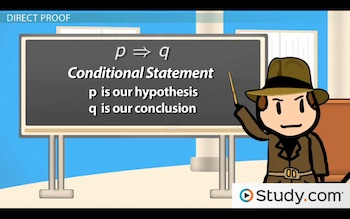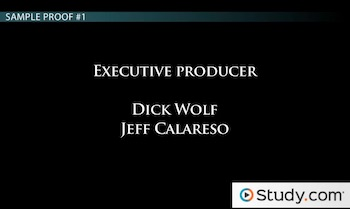# Direct Proofs: Definition and Applications

An error occurred trying to load this video.

Try refreshing the page, or contact customer support.

Coming up next: Geometric Proofs: Definition and Format

### You're on a roll. Keep up the good work!

Replay
Your next lesson will play in 10 seconds
• 0:01 Direct Proof
• 1:35 Sample Proof #1
• 4:03 Sample Proof #2
• 6:35 Lesson Summary
Save Save

Want to watch this again later?

Timeline
Autoplay
Autoplay
Speed Speed

#### Recommended Lessons and Courses for You

Lesson Transcript
Instructor: Jeff Calareso

Jeff teaches high school English, math and other subjects. He has a master's degree in writing and literature.

In math, we can't explain that something is true just because. That's not enough. In this lesson, we'll learn to prove things using one of our most straightforward tools, the direct proof.

## Direct Proof

In the Mathematics Justice System, the truth is represented by two separate, yet equally important groups: the math police, who investigate possible crimes against mathematics, and the direct proofs that determine whether statements are true. These are their stories.

That's right; it's time to be math police. You can be the rookie straight from the academy. I'll be the veteran cop trying to teach you a thing or two, though maybe you end up teaching me in the end.

Anyway, in this lesson, we're going to use direct proofs to get to the bottom of some math mysteries. A direct proof is a method of showing whether a conditional statement is true or false using known facts and rules.

A conditional statement is an 'if, then' statement. We might say if p, then q, where p is our hypothesis, and q is our conclusion. We can show this like this:We know p is true, but we need to find out if q is true.

You see conditional statements all the time. If you stay up too late, then you'll be tired tomorrow. Or if you give a mouse a cookie, then he'll want a glass of milk.

When we're completing direct proofs, our statements don't even need to include the words 'if' and 'then.' Consider this one: The product of any two odd integers is odd. How is that a statement we can prove? We could rephrase it as:

If x and y are odd integers, then x * y results in an odd integer.

Maybe we know we have a murder victim. We need to prove whether or not the shady husband did it.

## Sample Proof #1

Ok, but murder is outside of the math police's jurisdiction. With direct proofs, we're more likely to see something like this:

If a and b are both odd, then a + b is even.

So, our p is 'if a and b are both odd.' Our q is 'then a + b is even.' Our direct proof will be a series of statements that gets us from p to q. We need to fill in the gaps, like putting the pieces together when solving a murder.

Got your crime scene face on? Let's do this. We build our proof as a list, with each step on its own line.

First, we'll state, Suppose a and b are odd integers. That's the first half of our statement, or our p.

Next, we'll state, Then a = 2k + 1 and b = 2l + 1, where k and l are integers. Ok, rookie, are you with me? No? By definition, an even integer is just two times some integer. For example, 8 is just 2 * 4. And an odd integer is two times some integer, plus 1. Think of any odd integer, like 11. 11 is just 2 * 5, plus 1. So, we're picking variables for integers, k and l, and defining a and b in terms of them. If a is 11, then a is 2*5 + 1, right? Right.

Next, we can say, Therefore, a + b = (2k + 1) + (2l + 1) = 2(k + l + 1). We want to find out what a + b is - always keep your focus on the end goal. We just substituted here, then simplified.

Now we can say, If k and l are integers, so is k + l + 1. Why? We already know k and l are integers. What about 1? Yep, also an integer. So, the sum of three integers is also an integer.

Finally, we can say, a + b is even. That's what we wanted to prove. We just solved the case. Did you follow me? a + b must be even if it's equal to 2 times an integer. Remember earlier when we said that an even integer is just two times an integer? We just showed that a + b is equal to two times an integer, so this case is closed. Time for a doughnut and the closing credits:Yep, like that.

To unlock this lesson you must be a Study.com Member.

### Register to view this lesson

Are you a student or a teacher?

#### See for yourself why 30 million people use Study.com

##### Become a Study.com member and start learning now.
Back
What teachers are saying about Study.com

### Earning College Credit

Did you know… We have over 200 college courses that prepare you to earn credit by exam that is accepted by over 1,500 colleges and universities. You can test out of the first two years of college and save thousands off your degree. Anyone can earn credit-by-exam regardless of age or education level.